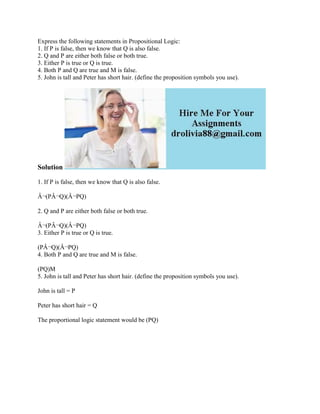Diese Präsentation wurde erfolgreich gemeldet.

# Express the following statements in Propositional Logic- 1- If P is fa.docx

Anzeige
Anzeige
Anzeige
Anzeige
Anzeige
Anzeige
Anzeige
Anzeige
Anzeige
Anzeige
Anzeige×

1 von 2 Anzeige

# Express the following statements in Propositional Logic- 1- If P is fa.docx

Express the following statements in Propositional Logic:
1. If P is false, then we know that Q is also false.
2. Q and P are either both false or both true.
3. Either P is true or Q is true.
4. Both P and Q are true and M is false.
5. John is tall and Peter has short hair. (define the proposition symbols you use).
Solution
1. If P is false, then we know that Q is also false.
Â¬(PÂ¬Q)(Â¬PQ)
2. Q and P are either both false or both true.
Â¬(PÂ¬Q)(Â¬PQ)
3. Either P is true or Q is true.
(PÂ¬Q)(Â¬PQ)
4. Both P and Q are true and M is false.
(PQ)M
5. John is tall and Peter has short hair. (define the proposition symbols you use).
John is tall = P
Peter has short hair = Q
The proportional logic statement would be (PQ)
.

Express the following statements in Propositional Logic:
1. If P is false, then we know that Q is also false.
2. Q and P are either both false or both true.
3. Either P is true or Q is true.
4. Both P and Q are true and M is false.
5. John is tall and Peter has short hair. (define the proposition symbols you use).
Solution
1. If P is false, then we know that Q is also false.
Â¬(PÂ¬Q)(Â¬PQ)
2. Q and P are either both false or both true.
Â¬(PÂ¬Q)(Â¬PQ)
3. Either P is true or Q is true.
(PÂ¬Q)(Â¬PQ)
4. Both P and Q are true and M is false.
(PQ)M
5. John is tall and Peter has short hair. (define the proposition symbols you use).
John is tall = P
Peter has short hair = Q
The proportional logic statement would be (PQ)
.

Anzeige
Anzeige

### Express the following statements in Propositional Logic- 1- If P is fa.docx

1. 1. Express the following statements in Propositional Logic: 1. If P is false, then we know that Q is also false. 2. Q and P are either both false or both true. 3. Either P is true or Q is true. 4. Both P and Q are true and M is false. 5. John is tall and Peter has short hair. (define the proposition symbols you use). Solution 1. If P is false, then we know that Q is also false. Â¬(PÂ¬Q)(Â¬PQ) 2. Q and P are either both false or both true. Â¬(PÂ¬Q)(Â¬PQ) 3. Either P is true or Q is true. (PÂ¬Q)(Â¬PQ) 4. Both P and Q are true and M is false. (PQ)M 5. John is tall and Peter has short hair. (define the proposition symbols you use). John is tall = P Peter has short hair = Q The proportional logic statement would be (PQ)